# The Sieve of Eratosthenes - Part 1

May. 15, 2022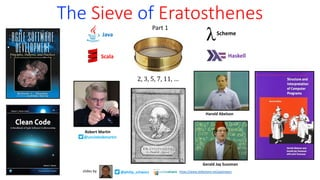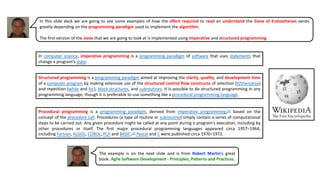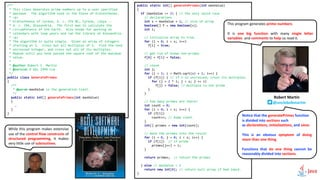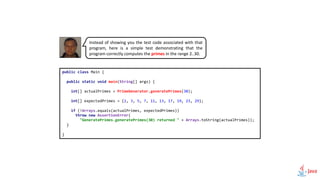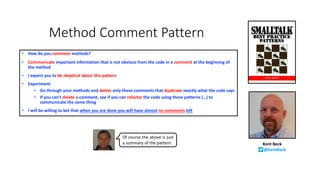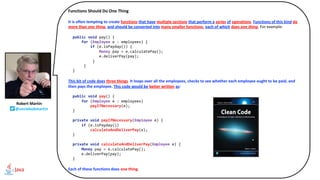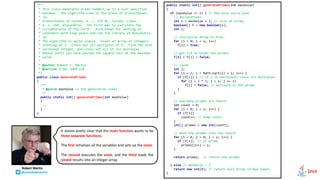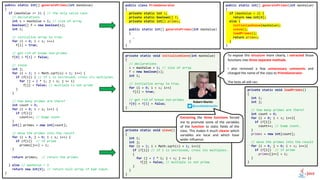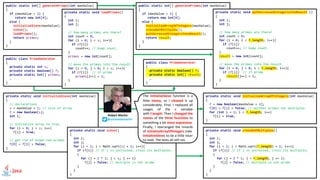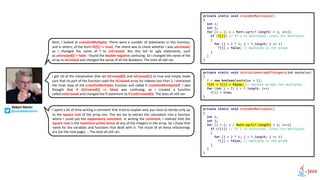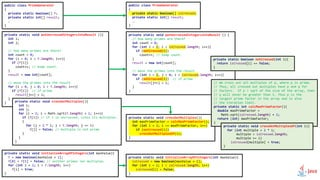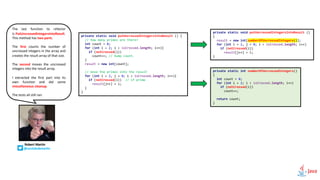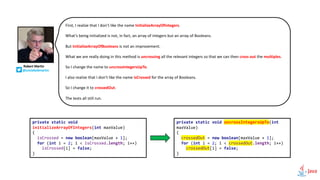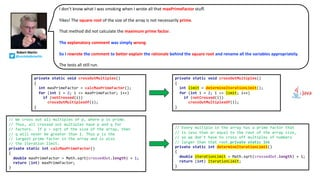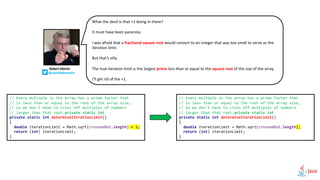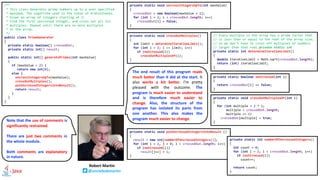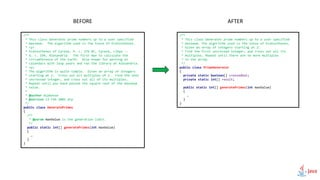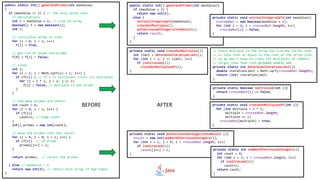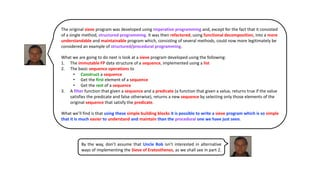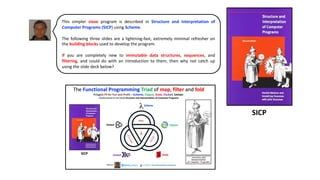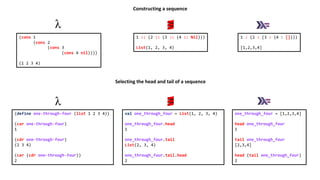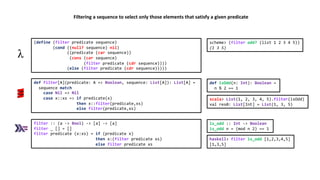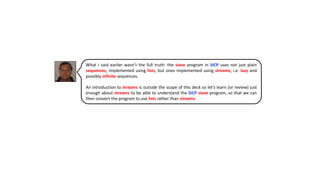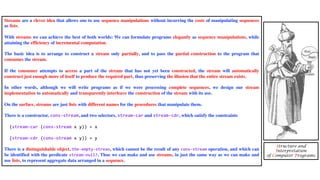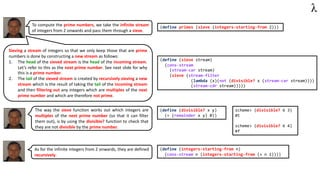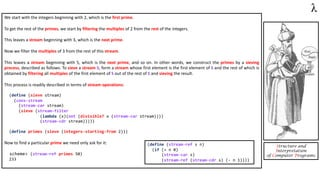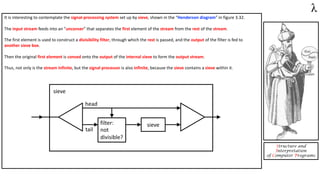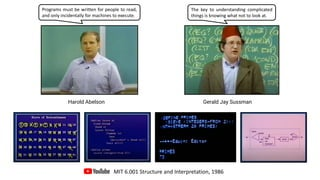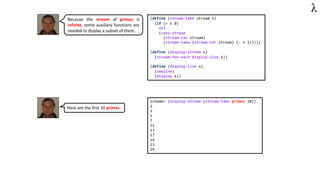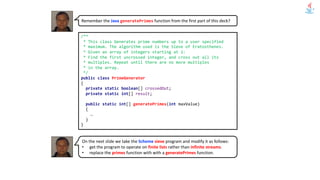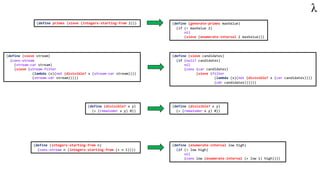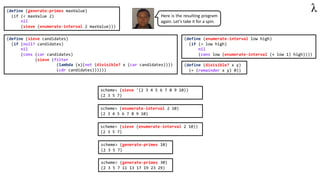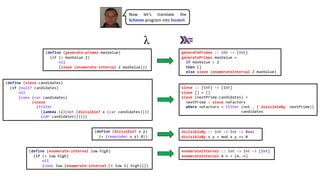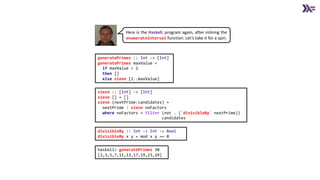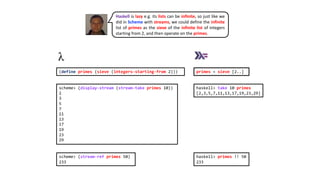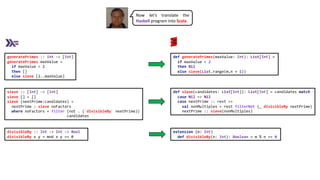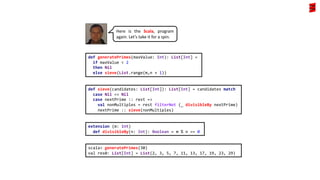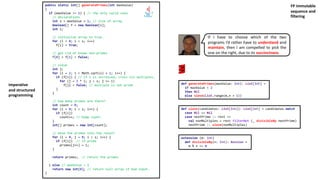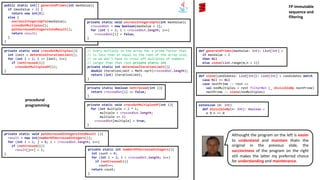1 of 50

### The Sieve of Eratosthenes - Part 1

1. The Sieve of Eratosthenes Part 1 Haskell Scala Scheme Java 2, 3, 5, 7, 11, … Robert Martin @unclebobmartin Harold Abelson Gerald Jay Sussman @philip_schwarz slides by https://www.slideshare.net/pjschwarz
2. In this slide deck we are going to see some examples of how the effort required to read an understand the Sieve of Eratosthenes varies greatly depending on the programming paradigm used to implement the algorithm. The first version of the sieve that we are going to look at is implemented using imperative and structured programming. The example is on the next slide and is from Robert Martin’s great book: Agile Software Development - Principles, Patterns and Practices. In computer science, imperative programming is a programming paradigm of software that uses statements that change a program's state. Structured programming is a programming paradigm aimed at improving the clarity, quality, and development time of a computer program by making extensive use of the structured control flow constructs of selection (if/then/else) and repetition (while and for), block structures, and subroutines. It is possible to do structured programming in any programming language, though it is preferable to use something like a procedural programming language. Procedural programming is a programming paradigm, derived from imperative programming, based on the concept of the procedure call. Procedures (a type of routine or subroutine) simply contain a series of computational steps to be carried out. Any given procedure might be called at any point during a program's execution, including by other procedures or itself. The first major procedural programming languages appeared circa 1957–1964, including Fortran, ALGOL, COBOL, PL/I and BASIC. Pascal and C were published circa 1970–1972.
3. public static int[] generatePrimes(int maxValue) { if (maxValue >= 2) { // the only valid case // declarations int s = maxValue + 1; // size of array boolean[] f = new boolean[s]; int i; // initialize array to true. for (i = 0; i < s; i++) f[i] = true; // get rid of known non-primes f = f = false; // sieve int j; for (i = 2; i < Math.sqrt(s) + 1; i++) { if (f[i]) { // if i is uncrossed, cross its multiples. for (j = 2 * i; j < s; j += i) f[j] = false; // multiple is not prime } } // how many primes are there? int count = 0; for (i = 0; i < s; i++) { if (f[i]) count++; // bump count. } int[] primes = new int[count]; // move the primes into the result for (i = 0, j = 0; i < s; i++) { if (f[i]) // if prime primes[j++] = i; } return primes; // return the primes } else // maxValue < 2 return new int; // return null array if bad input. } /** * This class Generates prime numbers up to a user specified * maximum. The algorithm used is the Sieve of Eratosthenes. * <p> * Eratosthenes of Cyrene, b. c. 276 BC, Cyrene, Libya -- * d. c. 194, Alexandria. The first man to calculate the * circumference of the Earth. Also known for working on * calendars with leap years and ran the library at Alexandria. * <p> * The algorithm is quite simple. Given an array of integers * starting at 2. Cross out all multiples of 2. Find the next * uncrossed integer, and cross out all of its multiples. * Repeat until you have passed the square root of the maximum * value. * * @author Robert C. Martin * @version 9 Dec 1999 rcm */ public class GeneratePrimes { /** * @param maxValue is the generation limit. */ public static int[] generatePrimes(int maxValue) { … } } While this program makes extensive use of the control flow constructs of structured programming, it makes very little use of subroutines. This program generates prime numbers. It is one big function with many single letter variables and comments to help us read it. Robert Martin @unclebobmartin Notice that the generatePrimes function is divided into sections such as declarations, initializations, and sieve. This is an obvious symptom of doing more than one thing. Functions that do one thing cannot be reasonably divided into sections.
4. public class Main { public static void main(String[] args) { int[] actualPrimes = PrimeGenerator.generatePrimes(30); int[] expectedPrimes = {2, 3, 5, 7, 11, 13, 17, 19, 23, 29}; if (!Arrays.equals(actualPrimes, expectedPrimes)) throw new AssertionError( "GeneratePrimes.generatePrimes(30) returned " + Arrays.toString(actualPrimes)); } } Instead of showing you the test code associated with that program, here is a simple test demonstrating that the program correctly computes the primes in the range 2..30.
5. I wrote the module … for the first XP Immersion. It was intended to be an example of bad coding and commenting style. Kent Beck then refactored this code into a much more pleasant form in front of several dozen enthusiastic students. Later I adapted the example for my book Agile Software Development, Principles, Patterns, and Practices and the first of my Craftsman articles published in Software Development magazine. What I find fascinating about this module is that there was a time when many of us would have considered it “well documented.” Now we see it as a small mess. See how many different comment problems you can find. Robert Martin @unclebobmartin
6. Jeff Langr @jlangr Commenting is more of an anti-pattern than anything else. Comments indicate that code is not communicating clearly. As others have said, "Comments are lies." You can't trust comments; the only thing you can truly depend on is working code. Strive to eliminate comments in your code. Martin Fowler @martinfowler Comments are often used as a deodorant for the rotten whiffs of bad code. When you feel the need to write a comment, first try to refactor the code so that any comment becomes superfluous. Robert Martin @unclebobmartin The proper use of comments is to compensate for our failure to express ourself in code. Note that I used the word failure. I meant it. Comments are always failures. We must have them because we cannot always figure out how to express ourselves without them, but their use is not a cause for celebration. So when you find yourself in a position where you need to write a comment, think it through and see whether there isn’t some way to turn the tables and express yourself in code. Every time you express yourself in code, you should pat yourself on the back. Every time you write a comment, you should grimace and feel the failure of your ability of expression.
7. Method Comment Pattern • How do you comment methods? • Communicate important information that is not obvious from the code in a comment at the beginning of the method • I expect you to be skeptical about this pattern • Experiment: • Go through your methods and delete only those comments that duplicate exactly what the code says • If you can’t delete a comment, see if you can refactor the code using these patterns (…) to communicate the same thing • I will be willing to bet that when you are done you will have almost no comments left Kent Beck @KentBeck Of course the above is just a summary of the pattern.
8. Functions Should Do One Thing It is often tempting to create functions that have multiple sections that perform a series of operations. Functions of this kind do more than one thing, and should be converted into many smaller functions, each of which does one thing. For example: public void pay() { for (Employee e : employees) { if (e.isPayday()) { Money pay = e.calculatePay(); e.deliverPay(pay); } } } This bit of code does three things. It loops over all the employees, checks to see whether each employee ought to be paid, and then pays the employee. This code would be better written as: public void pay() { for (Employee e : employees) payIfNecessary(e); } private void payIfNecessary(Employee e) { if (e.isPayday()) calculateAndDeliverPay(e); } private void calculateAndDeliverPay(Employee e) { Money pay = e.calculatePay(); e.deliverPay(pay); } Each of these functions does one thing. Robert Martin @unclebobmartin
9. Understand the Algorithm Lots of very funny code is written because people don’t take the time to understand the algorithm. They get something to work by plugging in enough if statements and flags, without really stopping to consider what is really going on. Programming is often an exploration. You think you know the right algorithm for something, but then you wind up fiddling with it, prodding and poking at it, until you get it to “work.” How do you know it “works”? Because it passes the test cases you can think of. There is nothing wrong with this approach. Indeed, often it is the only way to get a function to do what you think it should. However, it is not sufficient to leave the quotation marks around the word “work.” Before you consider yourself to be done with a function, make sure you understand how it works. It is not good enough that it passes all the tests. You must know10 that the solution is correct. Often the best way to gain this knowledge and understanding is to refactor the function into something that is so clean and expressive that it is obvious how it works. 10. There is a difference between knowing how the code works and knowing whether the algorithm will do the job required of it. Being unsure that an algorithm is appropriate is often a fact of life. Being unsure what your code does is just laziness. Robert Martin @unclebobmartin
10. The prime generator is hard to understand and maintain because it consists of a single method that does more than one thing. The method is divided into sections (each signposted by a comment), with each section doing one of the multiple things. On the next slide, Uncle Bob points asserts that if a program is difficult to understand and/or change then it is broken and needs fixing.
11. Robert Martin @unclebobmartin Every software module has three functions. First is the function it performs while executing. This function is the reason for the module’s existence. The second function of a module is to afford change. Almost all modules will change in the course of their lives, and it is the responsibility of the developers to make sure that such changes are as simple as possible to make. The third function of a module is to communicate to its readers. Developers who are not familiar with the module should be able to read and understand it without undue mental gymnastics. A module that is difficult to change is broken and needs fixing, even though it works. A module that does not communicate is broken and needs to be fixed. What does it take to make a module easy to read and easy to change? Much of this book is dedicated to principles and patterns whose primary goal is to help you create modules that are flexible and adaptable. But it takes something more than just principles and patterns to make a module that is easy to read and change. It takes attention. It takes discipline. It takes a passion for creating beauty.
12. What we are going to do next is follow Uncle Bob as he refactors the prime generator so that it becomes easier to understand and to change.
13. public static int[] generatePrimes(int maxValue) { if (maxValue >= 2) { // the only valid case // declarations int s = maxValue + 1; // size of array boolean[] f = new boolean[s]; int i; // initialize array to true. for (i = 0; i < s; i++) f[i] = true; // get rid of known non-primes f = f = false; // sieve int j; for (i = 2; i < Math.sqrt(s) + 1; i++) { if (f[i]) { // if i is uncrossed, cross its multiples. for (j = 2 * i; j < s; j += i) f[j] = false; // multiple is not prime } } // how many primes are there? int count = 0; for (i = 0; i < s; i++) { if (f[i]) count++; // bump count. } int[] primes = new int[count]; // move the primes into the result for (i = 0, j = 0; i < s; i++) { if (f[i]) // if prime primes[j++] = i; } return primes; // return the primes } else // maxValue < 2 return new int; // return null array if bad input. } /** * This class Generates prime numbers up to a user specified * maximum. The algorithm used is the Sieve of Eratosthenes. * <p> * Eratosthenes of Cyrene, b. c. 276 BC, Cyrene, Libya -- * d. c. 194, Alexandria. The first man to calculate the * circumference of the Earth. Also known for working on * calendars with leap years and ran the library at Alexandria. * <p> * The algorithm is quite simple. Given an array of integers * starting at 2. Cross out all multiples of 2. Find the next * uncrossed integer, and cross out all of its multiples. * Repeat until you have passed the square root of the maximum * value. * * @author Robert C. Martin * @version 9 Dec 1999 rcm */ public class GeneratePrimes { /** * @param maxValue is the generation limit. */ public static int[] generatePrimes(int maxValue) { … } } It seems pretty clear that the main function wants to be three separate functions. The first initializes all the variables and sets up the sieve. The second executes the sieve, and the third loads the sieved results into an integer array. Robert Martin @unclebobmartin
14. public static int[] generatePrimes(int maxValue) { if (maxValue >= 2) { // the only valid case // declarations int s = maxValue + 1; // size of array boolean[] f = new boolean[s]; int i; // initialize array to true. for (i = 0; i < s; i++) f[i] = true; // get rid of known non-primes f = f = false; // sieve int j; for (i = 2; i < Math.sqrt(s) + 1; i++) { if (f[i]) { // if i is uncrossed, cross its multiples. for (j = 2 * i; j < s; j += i) f[j] = false; // multiple is not prime } } // how many primes are there? int count = 0; for (i = 0; i < s; i++) { if (f[i]) count++; // bump count. } int[] primes = new int[count]; // move the primes into the result for (i = 0, j = 0; i < s; i++) { if (f[i]) // if prime primes[j++] = i; } return primes; // return the primes } else // maxValue < 2 return new int; // return null array if bad input. } public static int[] generatePrimes(int maxValue) { if (maxValue < 2) { return new int; else { initializeSieve(maxValue); sieve(); loadPrimes(); return primes; } } private static void initializeSieve(int maxValue) { // declarations s = maxValue + 1; // size of array f = new boolean[s]; int i; // initialize array to true. for (i = 0; i < s; i++) f[i] = true; // get rid of known non-primes f = f = false; } private static void sieve() { int i; int j; for (i = 2; i < Math.sqrt(s) + 1; i++){ if (f[i]) // if i is uncrossed, cross its multiples. { for (j = 2 * i; j < s; j += i) f[j] = false; // multiple is not prime } } } private static void loadPrimes() { int i; int j; // how many primes are there? int count = 0; for (i = 0; i < s; i++){ if (f[i]) count++; // bump count. } primes = new int[count]; // move the primes into the result for (i = 0, j = 0; i < s; i++){ if (f[i]) // if prime primes[j++] = i; } } public class PrimeGenerator { private static int s; private static boolean[] f; private static int[] primes; public static int[] generatePrimes(int maxValue) { … } } To expose this structure more clearly, I extracted those functions into three separate methods. I also removed a few unnecessary comments and changed the name of the class to PrimeGenerator. The tests all still ran. Robert Martin @unclebobmartin Extracting the three functions forced me to promote some of the variables of the function to static fields of the class. This makes it much clearer which variables are local and which have wider influence.
15. public class PrimeGenerator { private static int s; private static boolean[] f; private static int[] primes; … } public static int[] generatePrimes(int maxValue) { if (maxValue < 2) { return new int; else { initializeSieve(maxValue); sieve(); loadPrimes(); return primes; } } private static void initializeSieve(int maxValue) { // declarations s = maxValue + 1; // size of array f = new boolean[s]; int i; // initialize array to true. for (i = 0; i < s; i++) f[i] = true; // get rid of known non-primes f = f = false; } private static void sieve() { int i; int j; for (i = 2; i < Math.sqrt(s) + 1; i++){ if (f[i]) // if i is uncrossed, cross its multiples. { for (j = 2 * i; j < s; j += i) f[j] = false; // multiple is not prime } } } private static void loadPrimes() { int i; int j; // how many primes are there? int count = 0; for (i = 0; i < s; i++){ if (f[i]) count++; // bump count. } primes = new int[count]; // move the primes into the result for (i = 0, j = 0; i < s; i++){ if (f[i]) // if prime primes[j++] = i; } } private static void crossOutMultiples() { int i; int j; for (i = 2; i < Math.sqrt(f.length) + 1; i++){ if (f[i]) // if i is uncrossed, cross its multiples. { for (j = 2 * i; j < f.length; j += i) f[j] = false; // multiple is not prime } } } private static void initializeArrayOfIntegers(int maxValue) { f = new boolean[maxValue + 1]; f = f = false; // neither primes nor multiples for (int i = 2; i < f.length; i++) f[i] = true; } public static int[] generatePrimes(int maxValue) { if (maxValue < 2) { return new int; else { initializeArrayOfIntegers(maxValue); crossOutMultiples(); putUncrossedIntegersIntoResult(); return result; } } private static void putUncrossedIntegersIntoResult () { int i; int j; // how many primes are there? int count = 0; for (i = 0; i < f.length; i++){ if (f[i]) count++; // bump count. } result = new int[count]; // move the primes into the result for (i = 0, j = 0; i < f.length; i++){ if (f[i]) // if prime result[j++] = i; } } The InitializeSieve function is a little messy, so I cleaned it up considerably. First, I replaced all usages of the s variable with f.length. Then I changed the names of the three functions to something a bit more expressive. Finally, I rearranged the innards of InitializeArrayOfIntegers (née InitializeSieve) to be a little nicer to read. The tests all still ran. Robert Martin @unclebobmartin public class PrimeGenerator { private static boolean[] f; private static int[] result; … }
16. Next, I looked at crossOutMultiples. There were a number of statements in this function, and in others, of the form if(f[i] == true). The intent was to check whether i was uncrossed, so I changed the name of f to unCrossed. But this led to ugly statements, such as unCrossed[i] = false. I found the double negative confusing. So I changed the name of the array to isCrossed and changed the sense of all the Booleans. The tests all still ran. Robert Martin @unclebobmartin I got rid of the initialization that set isCrossed and isCrossed to true and simply made sure that no part of the function used the isCrossed array for indexes less than 2. I extracted the inner loop of the crossOutMultiples function and called it crossOutMultiplesOf. I also thought that if (isCrossed[i] == false) was confusing, so I created a function called notCrossed and changed the if statement to if (notCrossed(i)). The tests all still ran. I spent a bit of time writing a comment that tried to explain why you have to iterate only up to the square root of the array size. This led me to extract the calculation into a function where I could put the explanatory comment. In writing the comment, I realized that the square root is the maximum prime factor of any of the integers in the array. So I chose that name for the variables and functions that dealt with it. The result of all these refactorings are [on the next page] ... The tests all still ran. private static void crossOutMultiples() { int i; int j; for (i = 2; i < Math.sqrt(f.length) + 1; i++){ if (f[i]) // if i is uncrossed, cross its multiples. { for (j = 2 * i; j < f.length; j += i) f[j] = false; // multiple is not prime } } } private static void initializeArrayOfIntegers(int maxValue) { f = new boolean[maxValue + 1]; f = f = false; // neither primes nor multiples for (int i = 2; i < f.length; i++) f[i] = true; } private static void crossOutMultiples() { int i; int j; for (i = 2; i < Math.sqrt(f.length) + 1; i++){ if (f[i]) // if i is uncrossed, cross its multiples. { for (j = 2 * i; j < f.length; j += i) f[j] = false; // multiple is not prime } } }
17. public class PrimeGenerator { private static boolean[] f; private static int[] result; … } public class PrimeGenerator { private static boolean[] isCrossed; private static int[] result; … } private static void putUncrossedIntegersIntoResult (){ int i; int j; // how many primes are there? int count = 0; for (i = 0; i < f.length; i++){ if (f[i]) count++; // bump count. } result = new int[count]; // move the primes into the result for (i = 0, j = 0; i < f.length; i++){ if (f[i]) // if prime result[j++] = i; } } private static void putUncrossedIntegersIntoResult () { // how many primes are there? int count = 0; for (int i = 2; i < isCrossed.length; i++){ if (notCrossed(i)) count++; // bump count. } result = new int[count]; // move the primes into the result for (int i = 2, j = 0; i < isCrossed.length; i++){ if (notCrossed(i)) // if prime result[j++] = i; } } private static void crossOutMultiples(){ int i; int j; for (i = 2; i < Math.sqrt(f.length) + 1; i++){ if (f[i]) // if i is uncrossed, cross its multiples. { for (j = 2 * i; j < f.length; j += i) f[j] = false; // multiple is not prime } } } private static void crossOutMultiples(){ int maxPrimeFactor = calcMaxPrimeFactor(); for (int i = 2; i <= maxPrimeFactor; i++) if (notCrossed(i)) crossOutMultiplesOf(i); } // We cross out all multiples of p, where p is prime. // Thus, all crossed out multiples have p and q for // factors. If p > sqrt of the size of the array, then // q will never be greater than 1. Thus p is the // largest prime factor in the array and is also // the iteration limit. private static int calcMaxPrimeFactor(){ double maxPrimeFactor = Math.sqrt(isCrossed.length) + 1; return (int) maxPrimeFactor; } private static void crossOutMultiplesOf(int i){ for (int multiple = 2 * i; multiple < isCrossed.length; multiple += i) isCrossed[multiple] = true; } private static boolean notCrossed(int i){ return isCrossed[i] == false; } private static void initializeArrayOfIntegers(int maxValue){ f = new boolean[maxValue + 1]; f = f = false; // neither primes nor multiples for (int i = 2; i < f.length; i++) f[i] = true; } private static void initializeArrayOfIntegers(int maxValue){ isCrossed = new boolean[maxValue + 1]; for (int i = 2; i < isCrossed.length; i++) isCrossed[i] = false; }
18. The last function to refactor is PutUncrossedIntegersIntoResult. This method has two parts. The first counts the number of uncrossed integers in the array and creates the result array of that size. The second moves the uncrossed integers into the result array. I extracted the first part into its own function and did some miscellaneous cleanup. The tests all still ran. Robert Martin @unclebobmartin private static void putUncrossedIntegersIntoResult () { // how many primes are there? int count = 0; for (int i = 2; i < isCrossed.length; i++){ if (notCrossed(i)) count++; // bump count. } result = new int[count]; // move the primes into the result for (int i = 2, j = 0; i < isCrossed.length; i++){ if (notCrossed(i)) // if prime result[j++] = i; } } private static void putUncrossedIntegersIntoResult () { result = new int[numberOfUncrossedIntegers()]; for (int i = 2, j = 0; i < isCrossed.length; i++) if (notCrossed(i)) result[j++] = i; } private static int numberOfUncrossedIntegers() { int count = 0; for (int i = 2; i < isCrossed.length; i++) if (notCrossed(i)) count++; return count; }
19. Next, I made one final pass over the whole program, reading it from beginning to end, rather like one would read a geometric proof. This is an important step. So far, I’ve been refactoring fragments. Now I want to see whether the whole program hangs together as a readable whole. Robert Martin @unclebobmartin
20. Robert Martin @unclebobmartin First, I realize that I don’t like the name InitializeArrayOfIntegers. What’s being initialized is not, in fact, an array of integers but an array of Booleans. But InitializeArrayOfBooleans is not an improvement. What we are really doing in this method is uncrossing all the relevant integers so that we can then cross out the multiples. So I change the name to uncrossIntegersUpTo. I also realize that I don’t like the name isCrossed for the array of Booleans. So I change it to crossedOut. The tests all still run. private static void initializeArrayOfIntegers(int maxValue) { isCrossed = new boolean[maxValue + 1]; for (int i = 2; i < isCrossed.length; i++) isCrossed[i] = false; } private static void uncrossIntegersUpTo(int maxValue) { crossedOut = new boolean[maxValue + 1]; for (int i = 2; i < crossedOut.length; i++) crossedOut[i] = false; }
21. One might think that I’m being frivolous with these name changes, but with a refactoring browser, you can afford to do these kinds of tweaks; they cost virtually nothing. Even without a refactoring browser, a simple search and replace is pretty cheap. And the tests strongly mitigate any chance that we might unknowingly break something. Robert Martin @unclebobmartin
22. I don’t know what I was smoking when I wrote all that maxPrimeFactor stuff. Yikes! The square root of the size of the array is not necessarily prime. That method did not calculate the maximum prime factor. The explanatory comment was simply wrong. So I rewrote the comment to better explain the rationale behind the square root and rename all the variables appropriately. The tests all still run. Robert Martin @unclebobmartin private static void crossOutMultiples() { int maxPrimeFactor = calcMaxPrimeFactor(); for (int i = 2; i <= maxPrimeFactor; i++) if (notCrossed(i)) crossOutMultiplesOf(i); } // We cross out all multiples of p, where p is prime. // Thus, all crossed out multiples have p and q for // factors. If p > sqrt of the size of the array, then // q will never be greater than 1. Thus p is the // largest prime factor in the array and is also // the iteration limit. private static int calcMaxPrimeFactor() { double maxPrimeFactor = Math.sqrt(crossedOut.length) + 1; return (int) maxPrimeFactor; } private static void crossOutMultiples() { int limit = determineIterationLimit(); for (int i = 2; i <= limit; i++) if (notCrossed(i)) crossOutMultiplesOf(i); } // Every multiple in the array has a prime factor that // is less than or equal to the root of the array size, // so we don't have to cross off multiples of numbers // larger than that root.private static int private static int determineIterationLimit() { double iterationLimit = Math.sqrt(crossedOut.length) + 1; return (int) iterationLimit; }
23. // Every multiple in the array has a prime factor that // is less than or equal to the root of the array size, // so we don't have to cross off multiples of numbers // larger than that root.private static int private static int determineIterationLimit() { double iterationLimit = Math.sqrt(crossedOut.length) + 1; return (int) iterationLimit; } // Every multiple in the array has a prime factor that // is less than or equal to the root of the array size, // so we don't have to cross off multiples of numbers // larger than that root.private static int private static int determineIterationLimit() { double iterationLimit = Math.sqrt(crossedOut.length); return (int) iterationLimit; } Robert Martin @unclebobmartin What the devil is that +1 doing in there? It must have been paranoia. I was afraid that a fractional square root would convert to an integer that was too small to serve as the iteration limit. But that’s silly. The true iteration limit is the largest prime less than or equal to the square root of the size of the array. I’ll get rid of the +1.
24. The tests all run, but that last change makes me pretty nervous. I understand the rationale behind the square root, but I’ve got a nagging feeling that there may be some corner cases that aren’t being covered. So I’ll write another test that checks that there are no multiples in any of the prime lists between 2 and 500. … The new test passes, and my fears are allayed. The rest of the code reads pretty nicely. So I think we’re done. The final version is shown on the next slide. Robert Martin @unclebobmartin
25. /** * This class Generates prime numbers up to a user specified * maximum. The algorithm used is the Sieve of Eratosthenes. * Given an array of integers starting at 2: * Find the first uncrossed integer, and cross out all its * multiples. Repeat until there are no more multiples * in the array. */ public class PrimeGenerator { private static boolean[] crossedOut; private static int[] result; public static int[] generatePrimes(int maxValue) { if (maxValue < 2) { return new int; else { uncrossIntegersUpTo(maxValue); crossOutMultiples(); putUncrossedIntegersIntoResult(); return result; } } } private static void uncrossIntegersUpTo(int maxValue) { crossedOut = new boolean[maxValue + 1]; for (int i = 2; i < crossedOut.length; i++) crossedOut[i] = false; } private static void crossOutMultiples() { int limit = determineIterationLimit(); for (int i = 2; i <= limit; i++) if (notCrossed(i)) crossOutMultiplesOf(i); } // Every multiple in the array has a prime factor that // is less than or equal to the root of the array size, // so we don't have to cross off multiples of numbers // larger than that root.private static int private static int determineIterationLimit() { double iterationLimit = Math.sqrt(crossedOut.length); return (int) iterationLimit; } private static void putUncrossedIntegersIntoResult () { result = new int[numberOfUncrossedIntegers()]; for (int i = 2, j = 0; i < crossedOut.length; i++) if (notCrossed(i)) result[j++] = i; } private static int numberOfUncrossedIntegers() { int count = 0; for (int i = 2; i < crossedOut.length; i++) if (notCrossed(i)) count++; return count; } private static boolean notCrossed(int i) { return crossedOut[i] == false; } private static void crossOutMultiplesOf(int i) { for (int multiple = 2 * i; multiple < crossedOut.length; multiple += i) crossedOut[multiple] = true; } Robert Martin @unclebobmartin Note that the use of comments is significantly restrained. There are just two comments in the whole module. Both comments are explanatory in nature. The end result of this program reads much better than it did at the start. It also works a bit better. I’m pretty pleased with the outcome. The program is much easier to understand and is therefore much easier to change. Also, the structure of the program has isolated its parts from one another. This also makes the program much easier to change.
26. /** * This class Generates prime numbers up to a user specified * maximum. The algorithm used is the Sieve of Eratosthenes. * Given an array of integers starting at 2: * Find the first uncrossed integer, and cross out all its * multiples. Repeat until there are no more multiples * in the array. */ public class PrimeGenerator { private static boolean[] crossedOut; private static int[] result; public static int[] generatePrimes(int maxValue) { … } } /** * This class Generates prime numbers up to a user specified * maximum. The algorithm used is the Sieve of Eratosthenes. * <p> * Eratosthenes of Cyrene, b. c. 276 BC, Cyrene, Libya -- * d. c. 194, Alexandria. The first man to calculate the * circumference of the Earth. Also known for working on * calendars with leap years and ran the library at Alexandria. * <p> * The algorithm is quite simple. Given an array of integers * starting at 2. Cross out all multiples of 2. Find the next * uncrossed integer, and cross out all of its multiples. * Repeat until you have passed the square root of the maximum * value. * * @author Alphonse * @version 13 Feb 2002 atp */ public class GeneratePrimes { /** * @param maxValue is the generation limit. */ public static int[] generatePrimes(int maxValue) { … } } BEFORE AFTER
27. public static int[] generatePrimes(int maxValue){ if (maxValue < 2) { return new int; else { uncrossIntegersUpTo(maxValue); crossOutMultiples(); putUncrossedIntegersIntoResult(); return result; } } private static void uncrossIntegersUpTo(int maxValue){ crossedOut = new boolean[maxValue + 1]; for (int i = 2; i < crossedOut.length; i++) crossedOut[i] = false; } private static void crossOutMultiples(){ int limit = determineIterationLimit(); for (int i = 2; i <= limit; i++) if (notCrossed(i)) crossOutMultiplesOf(i); } // Every multiple in the array has a prime factor that // is less than or equal to the root of the array size, // so we don't have to cross off multiples of numbers // larger than that root.private static int private static int determineIterationLimit(){ double iterationLimit = Math.sqrt(crossedOut.length); return (int) iterationLimit; } private static void putUncrossedIntegersIntoResult (){ result = new int[numberOfUncrossedIntegers()]; for (int i = 2, j = 0; i < crossedOut.length; i++) if (notCrossed(i)) result[j++] = i; } private static int numberOfUncrossedIntegers(){ int count = 0; for (int i = 2; i < crossedOut.length; i++) if (notCrossed(i)) count++; return count; } private static boolean notCrossed(int i){ return crossedOut[i] == false; } private static void crossOutMultiplesOf(int i){ for (int multiple = 2 * i; multiple < crossedOut.length; multiple += i) crossedOut[multiple] = true; } public static int[] generatePrimes(int maxValue) { if (maxValue >= 2) { // the only valid case // declarations int s = maxValue + 1; // size of array boolean[] f = new boolean[s]; int i; // initialize array to true. for (i = 0; i < s; i++) f[i] = true; // get rid of known non-primes f = f = false; // sieve int j; for (i = 2; i < Math.sqrt(s) + 1; i++) { if (f[i]) { // if i is uncrossed, cross its multiples. for (j = 2 * i; j < s; j += i) f[j] = false; // multiple is not prime } } // how many primes are there? int count = 0; for (i = 0; i < s; i++) { if (f[i]) count++; // bump count. } int[] primes = new int[count]; // move the primes into the result for (i = 0, j = 0; i < s; i++) { if (f[i]) // if prime primes[j++] = i; } return primes; // return the primes } else // maxValue < 2 return new int; // return null array if bad input. } BEFORE AFTER
28. The original sieve program was developed using imperative programming and, except for the fact that it consisted of a single method, structured programming. It was then refactored, using functional decomposition, into a more understandable and maintainable program which, consisting of several methods, could now more legitimately be considered an example of structured/procedural programming. What we are going to do next is look at a sieve program developed using the following: 1. The immutable FP data structure of a sequence, implemented using a list 2. The basic sequence operations to • Construct a sequence • Get the first element of a sequence • Get the rest of a sequence 3. A filter function that given a sequence and a predicate (a function that given a value, returns true if the value satisfies the predicate and false otherwise), returns a new sequence by selecting only those elements of the original sequence that satisfy the predicate. What we’ll find is that using these simple building blocks it is possible to write a sieve program which is so simple that it is much easier to understand and maintain than the procedural one we have just seen. By the way, don’t assume that Uncle Bob isn’t interested in alternative ways of implementing the Sieve of Eratosthenes, as we shall see in part 2.
29. This simpler sieve program is described in Structure and Interpretation of Computer Programs (SICP) using Scheme. The following three slides are a lightning-fast, extremely minimal refresher on the building blocks used to develop the program. If you are completely new to immutable data structures, sequences, and filtering, and could do with an introduction to them, then why not catch up using the slide deck below? SICP
30. 1 :: (2 :: (3 :: (4 :: Nil))) List(1, 2, 3, 4) 1 : (2 : (3 : (4 : []))) [1,2,3,4] (cons 1 (cons 2 (cons 3 (cons 4 nil)))) (1 2 3 4) Constructing a sequence val one_through_four = List(1, 2, 3, 4) one_through_four.head 1 one_through_four.tail List(2, 3, 4) one_through_four.tail.head 2 one_through_four = [1,2,3,4] head one_through_four 1 tail one_through_four [2,3,4] head (tail one_through_four) 2 (define one-through-four (list 1 2 3 4)) (car one-through-four) 1 (cdr one-through-four) (2 3 4) (car (cdr one-through-four)) 2 Selecting the head and tail of a sequence
31. def filter[A](predicate: A => Boolean, sequence: List[A]): List[A] = sequence match case Nil => Nil case x::xs => if predicate(x) then x::filter(predicate,xs) else filter(predicate,xs) filter :: (a -> Bool) -> [a] -> [a] filter _ [] = [] filter predicate (x:xs) = if (predicate x) then x:(filter predicate xs) else filter predicate xs (define (filter predicate sequence) (cond ((null? sequence) nil) ((predicate (car sequence)) (cons (car sequence) (filter predicate (cdr sequence)))) (else (filter predicate (cdr sequence))))) Filtering a sequence to select only those elements that satisfy a given predicate scheme> (filter odd? (list 1 2 3 4 5)) (1 3 5) def isOdd(n: Int): Boolean = n % 2 == 1 is_odd :: Int -> Boolean is_odd n = (mod n 2) == 1 scala> List(1, 2, 3, 4, 5).filter(isOdd) val res0: List[Int] = List(1, 3, 5) haskell> filter is_odd [1,2,3,4,5] [1,3,5]
32. What I said earlier wasn’t the full truth: the sieve program in SICP uses not just plain sequences, implemented using lists, but ones implemented using streams, i.e. lazy and possibly infinite sequences. An introduction to streams is outside the scope of this deck so let’s learn (or review) just enough about streams to be able to understand the SICP sieve program, so that we can then convert the program to use lists rather than streams.
33. Streams are a clever idea that allows one to use sequence manipulations without incurring the costs of manipulating sequences as lists. With streams we can achieve the best of both worlds: We can formulate programs elegantly as sequence manipulations, while attaining the efficiency of incremental computation. The basic idea is to arrange to construct a stream only partially, and to pass the partial construction to the program that consumes the stream. If the consumer attempts to access a part of the stream that has not yet been constructed, the stream will automatically construct just enough more of itself to produce the required part, thus preserving the illusion that the entire stream exists. In other words, although we will write programs as if we were processing complete sequences, we design our stream implementation to automatically and transparently interleave the construction of the stream with its use. On the surface, streams are just lists with different names for the procedures that manipulate them. There is a constructor, cons-stream, and two selectors, stream-car and stream-cdr, which satisfy the constraints (stream-car (cons-stream x y)) = x (stream-cdr (cons-stream x y)) = y There is a distinguishable object, the-empty-stream, which cannot be the result of any cons-stream operation, and which can be identified with the predicate stream-null?. Thus we can make and use streams, in just the same way as we can make and use lists, to represent aggregate data arranged in a sequence. Structure and Interpretation of Computer Programs
34. (define primes (sieve (integers-starting-from 2))) To compute the prime numbers, we take the infinite stream of integers from 2 onwards and pass them through a sieve. (define (sieve stream) (cons-stream (stream-car stream) (sieve (stream-filter (lambda (x)(not (divisible? x (stream-car stream)))) (stream-cdr stream))))) Sieving a stream of integers so that we only keep those that are prime numbers is done by constructing a new stream as follows: 1. The head of the sieved stream is the head of the incoming stream. Let’s refer to this as the next prime number. See next slide for why this is a prime number. 2. The tail of the sieved stream is created by recursively sieving a new stream which is the result of taking the tail of the incoming stream and then filtering out any integers which are multiples of the next prime number and which are therefore not prime. The way the sieve function works out which integers are multiples of the next prime number (so that it can filter them out), is by using the divisible? function to check that they are not divisible by the prime number. (define (divisible? x y) (= (remainder x y) 0)) scheme> (divisible? 6 3) #t scheme> (divisible? 6 4) #f (define (integers-starting-from n) (cons-stream n (integers-starting-from (+ n 1)))) As for the infinite integers from 2 onwards, they are defined recursively.
35. We start with the integers beginning with 2, which is the first prime. To get the rest of the primes, we start by filtering the multiples of 2 from the rest of the integers. This leaves a stream beginning with 3, which is the next prime. Now we filter the multiples of 3 from the rest of this stream. This leaves a stream beginning with 5, which is the next prime, and so on. In other words, we construct the primes by a sieving process, described as follows: To sieve a stream S, form a stream whose first element is the first element of S and the rest of which is obtained by filtering all multiples of the first element of S out of the rest of S and sieving the result. This process is readily described in terms of stream operations: (define (sieve stream) (cons-stream (stream-car stream) (sieve (stream-filter (lambda (x)(not (divisible? x (stream-car stream)))) (stream-cdr stream))))) (define primes (sieve (integers-starting-from 2))) Now to find a particular prime we need only ask for it: scheme> (stream-ref primes 50) 233 Structure and Interpretation of Computer Programs (define (stream-ref s n) (if (= n 0) (stream-car s) (stream-ref (stream-cdr s) (- n 1))))
36. It is interesting to contemplate the signal-processing system set up by sieve, shown in the “Henderson diagram” in figure 3.32. The input stream feeds into an ”unconser” that separates the first element of the stream from the rest of the stream. The first element is used to construct a divisibility filter, through which the rest is passed, and the output of the filter is fed to another sieve box. Then the original first element is consed onto the output of the internal sieve to form the output stream. Thus, not only is the stream infinite, but the signal processor is also infinite, because the sieve contains a sieve within it. Structure and Interpretation of Computer Programs filter: not divisible? sieve tail head sieve
37. The key to understanding complicated things is knowing what not to look at. Programs must be written for people to read, and only incidentally for machines to execute. Harold Abelson Gerald Jay Sussman MIT 6.001 Structure and Interpretation, 1986
38. scheme> (display-stream (stream-take primes 10)) 2 3 5 7 11 13 17 19 23 29 (define (stream-take stream n) (if (= n 0) nil (cons-stream (stream-car stream) (stream-take (stream-cdr stream) (- n 1))))) (define (display-stream s) (stream-for-each display-line s)) (define (display-line x) (newline) (display x)) Because the stream of primes is infinite, some auxiliary functions are needed to display a subset of them. Here are the first 10 primes.
39. Remember the Java generatePrimes function from the first part of this deck? /** * This class Generates prime numbers up to a user specified * maximum. The algorithm used is the Sieve of Eratosthenes. * Given an array of integers starting at 2: * Find the first uncrossed integer, and cross out all its * multiples. Repeat until there are no more multiples * in the array. */ public class PrimeGenerator { private static boolean[] crossedOut; private static int[] result; public static int[] generatePrimes(int maxValue) { … } } On the next slide we take the Scheme sieve program and modify it as follows: • get the program to operate on finite lists rather than infinite streams. • replace the primes function with with a generatePrimes function.
40. (define primes (sieve (integers-starting-from 2))) (define (sieve stream) (cons-stream (stream-car stream) (sieve (stream-filter (lambda (x)(not (divisible? x (stream-car stream)))) (stream-cdr stream))))) (define (divisible? x y) (= (remainder x y) 0)) (define (integers-starting-from n) (cons-stream n (integers-starting-from (+ n 1)))) (define (sieve candidates) (if (null? candidates) nil (cons (car candidates) (sieve (filter (lambda (x)(not (divisible? x (car candidates)))) (cdr candidates)))))) (define (generate-primes maxValue) (if (< maxValue 2) nil (sieve (enumerate-interval 2 maxValue))) (define (enumerate-interval low high) (if (> low high) nil (cons low (enumerate-interval (+ low 1) high)))) (define (divisible? x y) (= (remainder x y) 0))
41. scheme> (sieve '(2 3 4 5 6 7 8 9 10)) (2 3 5 7) (define (sieve candidates) (if (null? candidates) nil (cons (car candidates) (sieve (filter (lambda (x)(not (divisible? x (car candidates)))) (cdr candidates)))))) (define (generate-primes maxValue) (if (< maxValue 2) nil (sieve (enumerate-interval 2 maxValue))) scheme> (enumerate-interval 2 10) (2 3 4 5 6 7 8 9 10) (define (enumerate-interval low high) (if (> low high) nil (cons low (enumerate-interval (+ low 1) high)))) (define (divisible? x y) (= (remainder x y) 0)) scheme> (generate-primes 30) (2 3 5 7 11 13 17 19 23 29) scheme> (sieve (enumerate-interval 2 10)) (2 3 5 7) scheme> (generate-primes 10) (2 3 5 7) Here is the resulting program again. Let’s take it for a spin.
42. Now let’s translate the Scheme program into Haskell. (define (sieve candidates) (if (null? candidates) nil (cons (car candidates) (sieve (filter (lambda (x)(not (divisible? x (car candidates)))) (cdr candidates)))))) (define (generate-primes maxValue) (if (< maxValue 2) nil (sieve (enumerate-interval 2 maxValue))) (define (enumerate-interval low high) (if (> low high) nil (cons low (enumerate-interval (+ low 1) high)))) (define (divisible? x y) (= (remainder x y) 0)) generatePrimes :: Int -> [Int] generatePrimes maxValue = if maxValue < 2 then [] else sieve (enumerateInterval 2 maxValue) sieve :: [Int] -> [Int] sieve [] = [] sieve (nextPrime:candidates) = nextPrime : sieve noFactors where noFactors = filter (not . (`divisibleBy` nextPrime)) candidates divisibleBy :: Int -> Int -> Bool divisibleBy x y = mod x y == 0 enumerateInterval :: Int -> Int -> [Int] enumerateInterval m n = [m..n]
43. generatePrimes :: Int -> [Int] generatePrimes maxValue = if maxValue < 2 then [] else sieve [2..maxValue] sieve :: [Int] -> [Int] sieve [] = [] sieve (nextPrime:candidates) = nextPrime : sieve noFactors where noFactors = filter (not . (`divisibleBy` nextPrime)) candidates divisibleBy :: Int -> Int -> Bool divisibleBy x y = mod x y == 0 Here is the Haskell, program again, after inlining the enumerateInterval function. Let’s take it for a spin. haskell> generatePrimes 30 [2,3,5,7,11,13,17,19,23,29]
44. haskell> take 10 primes [2,3,5,7,11,13,17,19,23,29] haskell> primes !! 50 233 Haskell is lazy e.g. its lists can be infinite, so just like we did in Scheme with streams, we could define the infinite list of primes as the sieve of the infinite list of integers starting from 2, and then operate on the primes. primes = sieve [2..] (define primes (sieve (integers-starting-from 2))) scheme> (stream-ref primes 50) 233 scheme> (display-stream (stream-take primes 10)) 2 3 5 7 11 13 17 19 23 29
45. extension (m: Int) def divisibleBy(n: Int): Boolean = m % n == 0 def generatePrimes(maxValue: Int): List[Int] = if maxValue < 2 then Nil else sieve(List.range(m,n + 1)) def sieve(candidates: List[Int]): List[Int] = candidates match case Nil => Nil case nextPrime :: rest => val nonMultiples = rest filterNot (_ divisibleBy nextPrime) nextPrime :: sieve(nonMultiples) Now let’s translate the Haskell program into Scala. generatePrimes :: Int -> [Int] generatePrimes maxValue = if maxValue < 2 then [] else sieve [2..maxValue] sieve :: [Int] -> [Int] sieve [] = [] sieve (nextPrime:candidates) = nextPrime : sieve noFactors where noFactors = filter (not . (`divisibleBy` nextPrime)) candidates divisibleBy :: Int -> Int -> Bool divisibleBy x y = mod x y == 0
46. Here is the Scala, program again. Let’s take it for a spin. extension (m: Int) def divisibleBy(n: Int): Boolean = m % n == 0 def generatePrimes(maxValue: Int): List[Int] = if maxValue < 2 then Nil else sieve(List.range(m,n + 1)) def sieve(candidates: List[Int]): List[Int] = candidates match case Nil => Nil case nextPrime :: rest => val nonMultiples = rest filterNot (_ divisibleBy nextPrime) nextPrime :: sieve(nonMultiples) scala> generatePrimes(30) val res0: List[Int] = List(2, 3, 5, 7, 11, 13, 17, 19, 23, 29)
47. In the next two slides, let’s compare the Scala program with the Java program, both before and after refactoring the latter.
48. extension (m: Int) def divisibleBy(n: Int): Boolean = m % n == 0 def generatePrimes(maxValue: Int): List[Int] = if maxValue < 2 then Nil else sieve(List.range(m,n + 1)) def sieve(candidates: List[Int]): List[Int] = candidates match case Nil => Nil case nextPrime :: rest => val nonMultiples = rest filterNot (_ divisibleBy nextPrime) nextPrime :: sieve(nonMultiples) public static int[] generatePrimes(int maxValue) { if (maxValue >= 2) { // the only valid case // declarations int s = maxValue + 1; // size of array boolean[] f = new boolean[s]; int i; // initialize array to true. for (i = 0; i < s; i++) f[i] = true; // get rid of known non-primes f = f = false; // sieve int j; for (i = 2; i < Math.sqrt(s) + 1; i++) { if (f[i]) { // if i is uncrossed, cross its multiples. for (j = 2 * i; j < s; j += i) f[j] = false; // multiple is not prime } } // how many primes are there? int count = 0; for (i = 0; i < s; i++) { if (f[i]) count++; // bump count. } int[] primes = new int[count]; // move the primes into the result for (i = 0, j = 0; i < s; i++) { if (f[i]) // if prime primes[j++] = i; } return primes; // return the primes } else // maxValue < 2 return new int; // return null array if bad input. } If I have to choose which of the two programs I’d rather have to understand and maintain, then I am compelled to pick the one on the right, due to its succinctness. imperative and structured programming FP immutable sequence and filtering
49. public static int[] generatePrimes(int maxValue){ if (maxValue < 2) { return new int; else { uncrossIntegersUpTo(maxValue); crossOutMultiples(); putUncrossedIntegersIntoResult(); return result; } } private static void uncrossIntegersUpTo(int maxValue){ crossedOut = new boolean[maxValue + 1]; for (int i = 2; i < crossedOut.length; i++) crossedOut[i] = false; } private static void crossOutMultiples(){ int limit = determineIterationLimit(); for (int i = 2; i <= limit; i++) if (notCrossed(i)) crossOutMultiplesOf(i); } // Every multiple in the array has a prime factor that // is less than or equal to the root of the array size, // so we don't have to cross off multiples of numbers // larger than that root.private static int private static int determineIterationLimit(){ double iterationLimit = Math.sqrt(crossedOut.length); return (int) iterationLimit; } private static void putUncrossedIntegersIntoResult (){ result = new int[numberOfUncrossedIntegers()]; for (int i = 2, j = 0; i < crossedOut.length; i++) if (notCrossed(i)) result[j++] = i; } private static int numberOfUncrossedIntegers(){ int count = 0; for (int i = 2; i < crossedOut.length; i++) if (notCrossed(i)) count++; return count; } private static boolean notCrossed(int i){ return crossedOut[i] == false; } private static void crossOutMultiplesOf(int i){ for (int multiple = 2 * i; multiple < crossedOut.length; multiple += i) crossedOut[multiple] = true; } extension (m: Int) def divisibleBy(n: Int): Boolean = m % n == 0 def generatePrimes(maxValue: Int): List[Int] = if maxValue < 2 then Nil else sieve(List.range(m,n + 1)) def sieve(candidates: List[Int]): List[Int] = candidates match case Nil => Nil case nextPrime :: rest => val nonMultiples = rest filterNot (_ divisibleBy nextPrime) nextPrime :: sieve(nonMultiples) Althought the program on the left is easier to understand and maintain thatn the original in the previous slide, the succinctness of the program on the right still makes the latter my preferred choice for understanding and maintenance. procedural programming FP immutable sequence and filtering
50. That’s all for Part 1. See you in Part 2.